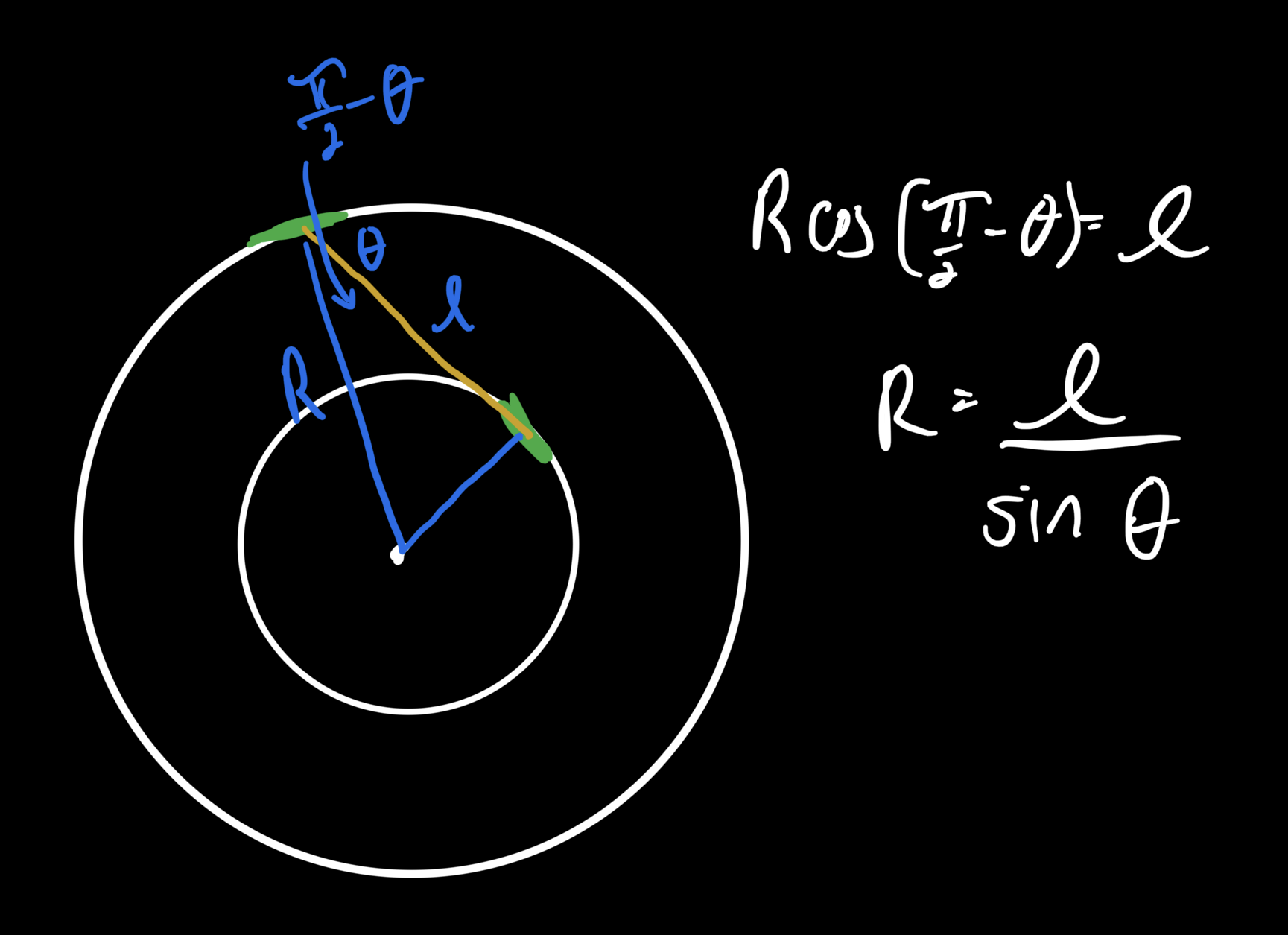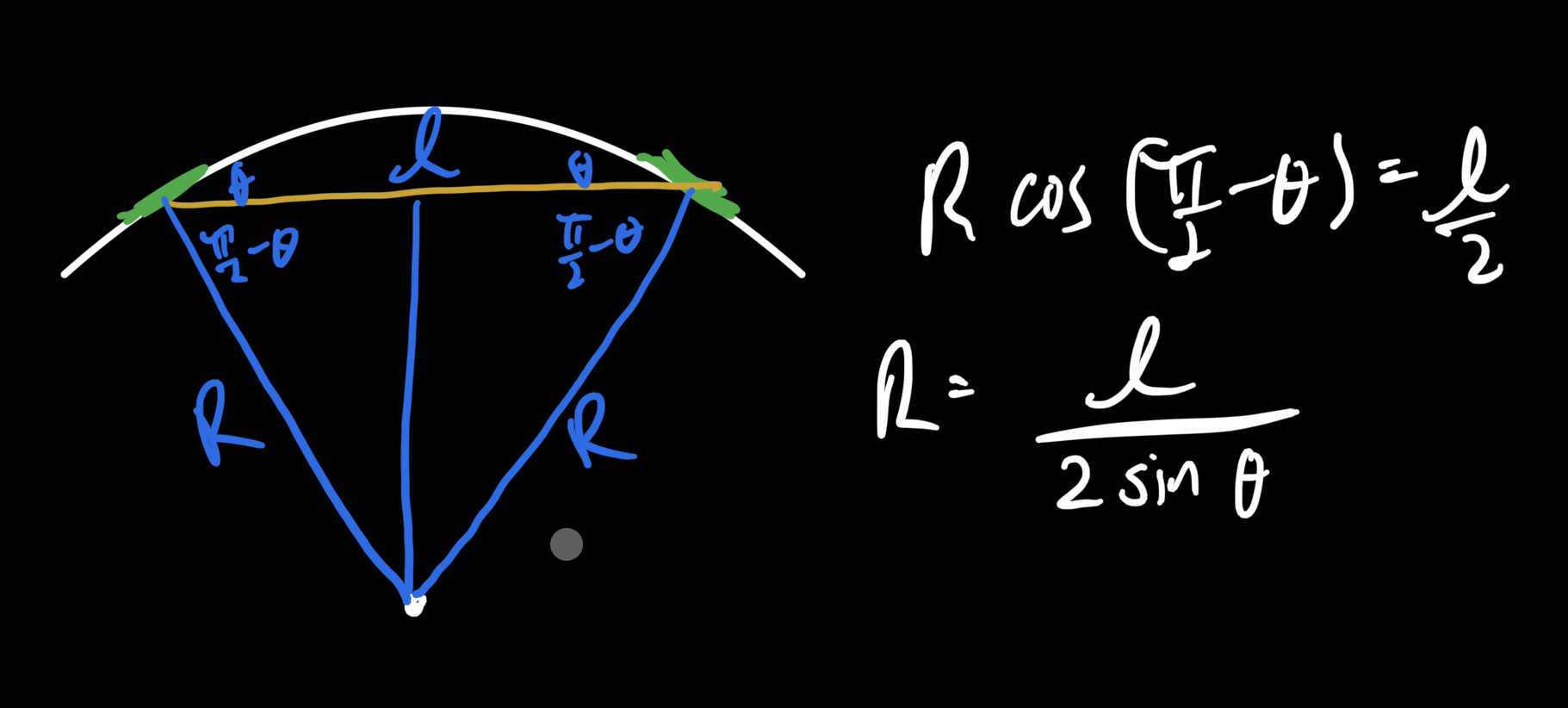Question: You’re driving your truck — which has the footprint of a bicycle — down to the grocery store to pick up yams for the big day. Boy do you love yourself some yams! When you’re halfway there, you realize you left your N-95 face mask at home. What a klutz! You make a U-turn, pointing holding your front tire $30\,^\circ$ to the left until you turn around.

When you’re almost home, you stroke your chin, contemplating your own forgetfulness, when you realize the mask has been on your face the whole time! Always one to outdo yourself, you decide that for your next U-turn, you’re going to angle the back tire a full $30\,^\circ$ of its own!

What are your turning radii in either case?

## Solution

### A few principles

The motion of the truck abides two basic rules:

• each tire points tangential to its current path
• with wheels fixed, the situation is not changed by how we look at it, so the truck travels in a circle

### Situation 1

The body of the truck points from one tire to the other. Since the back tire is parallel to the truck’s body, and each tire is tangent to the circle it’s on, the front tire can’t be on the same circle as the back one. Because the wheels are locked during motion, the circles have the same center.

To find the larger circle, we can project out a truck length away from the back tire $\ell,$ and wherever the front tire lands determines the outer circle which determines the turning radius.The small and large radii form right angles their respective tires, so $\ell$ is the projection of $R$ at the angle $\left(\pi/2 - \theta\right)$ and

\begin{align} R\times \cos\left(\pi/2 - \theta\right) &= \ell \\ R\times\sin\theta &= \ell \\ R &= \dfrac{\ell}{\sin\theta} \\ &= \dfrac{\ell}{\sin\pi/3} \end{align}

### Situation 2

This situation is simpler.

First of all, the two tires have to tilt in opposite directions relative to the body of the truck. Consider: if they tilted in the same direction, they’d be parallel and the truck would move on a straight line (circle of radius infinity).

The tightest circle they can manage is by pointing the full $30^\circ$ in opposing directions. And because they have the same angle, they’ll be on the same circle.From the drawing, half the length of the truck $\ell/2$ is the projection of $R$ at the angle $\left(\pi/2-\theta\right),$ so

\begin{align} R\times\cos\left(\pi/2 - \theta\right) &= \ell/2 \\ R\times\sin\theta &= \ell/2 \\ R &= \dfrac{\ell}{2\sin\theta} \\ &= \dfrac{\ell}{2\sin\pi/3} \end{align}

### General case

In general, the front and back tries can be at whatever angles $\phi$ and $\psi$ that we please, in which case $\ell$ is the sum of the cosine projections of the large and small radii:

$\ell = R\sin\phi + r\sin\psi$

but the small radius $r$ is related to $R$ by $r = \cos\phi \times R/\cos\psi,$ so

\begin{align} \left(\sin\phi + \sin\psi\cos\phi/\cos\psi\right) \times R &= \ell \\ R &= \dfrac{\ell}{\sin\phi + \sin\psi\cos\phi/\cos\psi} \\ &= \dfrac{\ell\cos\psi}{\sin\phi\cos\psi + \sin\psi\cos\phi} \\ &= \dfrac{\cos\psi}{\sin\left(\phi+\psi\right)}\times\ell \end{align}

which reduces to $\ell/\sin\phi$ when we plug in $\psi=0$ and to $\ell/\left(2\sin\phi\right)$ when $\psi=\phi.$